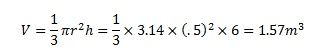# How to Find the Volume of a Cone

## Cone – Definition

A cone is a pyramid with a circular cross section. Therefore, its base is also circular. It can also be considered as a limiting case of a pyramid with infinite sides. The cone is a right cone if the apex (vertex) is directly above the center of the base and the perpendicular height h between the base and the apex passes through the center of the base. If the apex is offset from the center of the base, the cone is known as an oblique cone.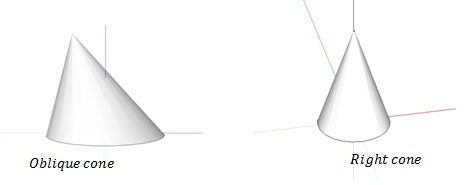## How to find the volume of a cone

For a cone with radius of base r and height h the volume can be obtained by the formula,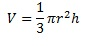The result holds for both oblique and right cones. The result is derived as following (in this case only the right cone is considered, and the geometry of the oblique cone is a little more complex than the right cone. However, the same results can be obtained independent of the position of the apex):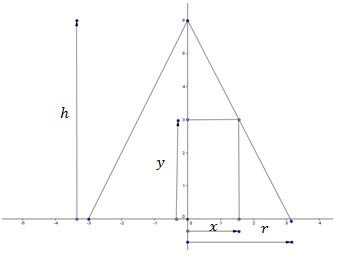Consider a cone with base radius r and perpendicular height h, with the center of the based positioned at the origin. If an incremental distance in the y direction is given by dy, the incremental volume in that direction will be a circular slab with thickness dy and radius x. Therefore, dv=πx2dy
From the geometry of the cone,(taking the gradient of the slope gives)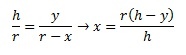The integral gives the volume of the cone,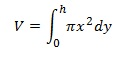Substituting for x gives,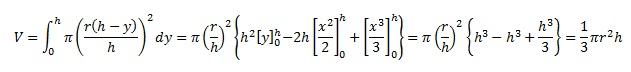## Find the volume of a cone – Examples

• A right cone has a radius of 10cm at the base and a perpendicular height of 30cm. Calculate the volume occupied by the cone. Radius (r) is 10cm and height is 30cm. Therefore, the volume is,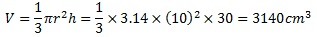• An oblique cone has a diameter of a 1m. If the perpendicular height is 6m find the volume of the cone.